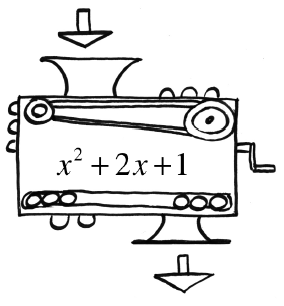### Home > A2C > Chapter 1 > Lesson 1.2.1 > Problem1-75

1-75.If the number $1$ is the output for Carmichael’s function machine shown at right, how can you find out what number was dropped in? Find the number(s) that could have been dropped in.

Since $1$ is the output, set the expression inside of the function machine equal to $1$.

$x^2+2x+1=1$

$x^2+2x=0$

$x(x+2)=0$

Use the Zero Product Property: $x=0,x=−2$